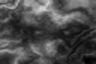# WebGLFundamentals.org

Fix, Fork, Contribute

# How to import a heightmap in WebGL

## Question:

I know that in theory you have to first find the coordinates on the height map like (x = `width HM / width Terrain * x Terrain`) and y coordinate (`y = height HM / height Terrain * y Terrain`) and after getting the location on the height map, we get the actual height by `min_height + (colorVal / (max_color - min_color) * *max_height - min_height`) thus returning a Z value for a particular segment.

But how can i actually import the height map and take its parameters? I'm writing in javascript with no additional libraries (three,babylon).

edit

Currently i'm hardcoding the z values based on x and y ranges:

``````    Plane.prototype.modifyGeometry=function(x,y){
if((x>=0&&x<100)&&(y>=0&&y<100)){
return 25;
}
else if((x>=100&&x<150)&&(y>=100&&y<150)){
return 20;
}
else if((x>=150&&x<200)&&(y>=150&&y<200)){
return 15;
}
else if((x>=200&&x<250)&&(y>=200&&y<250)){
return 10;
}
else if((x>=250&&x<300)&&(y>=250&&y<300)){
return 5;
}
else{
return 0;
}
``````

edit

i can get a flat grid (or with randomly generated heights), but as soon as i add the image data, i get a blank screen(no errors though). Here is the code (i changed it up a bit):

``````var gl;
var canvas;

var img = new Image();
img.crossOrigin = 'anonymous';
img.src = 'https://threejsfundamentals.org/threejs/resources/images/heightmap-96x64.png';

var gridWidth;
var gridDepth;
var gridPoints = [];
var gridIndices = [];
var rowOff = 0;
var rowStride = gridWidth + 1;
var numVertices = (gridWidth * 2 * (gridDepth + 1)) + (gridDepth * 2 * (gridWidth + 1));

//creating plane
function generateHeightPoints() {

var ctx = document.createElement("canvas").getContext("2d"); //using 2d canvas to read image
ctx.canvas.width = img.width;
ctx.canvas.height = img.height;
ctx.drawImage(img, 0, 0);
var imgData = ctx.getImageData(0, 0, ctx.canvas.width, ctx.canvas.height);

gridWidth = imgData.width - 1;
gridDepth = imgData.height - 1;

for (var z = 0; z <= gridDepth; ++z) {
for (var x = 0; x <= gridWidth; ++x) {
var offset = (z * imgData.width + x) * 4;
var height = imgData.data[offset] * 10 / 255;
gridPoints.push(x, height, z);
}
}
}

function generateIndices() {
for (var z = 0; z<=gridDepth; ++z) {
rowOff = z*rowStride;
for(var x = 0; x<gridWidth; ++x) {
gridIndices.push(rowOff+x,rowOff+x+1);
}
}

for (var x = 0; x<=gridWidth; ++x) {
for(var z = 0; z<gridDepth; ++z) {
rowOff = z * rowStride;
gridIndices.push(rowOff+x,rowOff+x+rowStride);
}
}
}
//init

//program init
{
canvas = document.getElementById( "gl-canvas" );

gl = WebGLUtils.setupWebGL( canvas );
if ( !gl ) { alert( "WebGL isn't available" ); }

gl.viewport(0, 0, gl.canvas.width, gl.canvas.height);

gl.useProgram( program );

generateHeightPoints();
generateIndices();

var positionBuffer = gl.createBuffer();
gl.bindBuffer(gl.ARRAY_BUFFER, positionBuffer);
gl.bufferData(gl.ARRAY_BUFFER, new Float32Array(gridPoints),
gl.STATIC_DRAW);

var indexBuffer = gl.createBuffer();
gl.bindBuffer(gl.ELEMENT_ARRAY_BUFFER, indexBuffer);
gl.bufferData(gl.ELEMENT_ARRAY_BUFFER, new Uint16Array(gridIndices),
gl.STATIC_DRAW);

var vPosition = gl.getAttribLocation( program, "vPosition" );
gl.vertexAttribPointer( vPosition, 3, gl.FLOAT, false, 0, 0 );
gl.enableVertexAttribArray( vPosition );

var matrixLoc = gl.getUniformLocation(program, 'matrix');

var m4 = twgl.m4;
var projection = m4.perspective(60 * Math.PI / 180, gl.canvas.clientWidth /
gl.canvas.clientHeight, 0.1, 100);
var cameraPosition = [-18, 15, -10];
var target = [gridWidth / 2, -10, gridDepth / 2];
var up = [0, 1, 0];
var camera = m4.lookAt(cameraPosition, target, up);
var view = m4.inverse(camera);
var mat = m4.multiply(projection, view);

gl.uniformMatrix4fv(matrixLoc, false, mat);

render();

}

function render() {

gl.drawElements(gl.LINES, numVertices, gl.UNSIGNED_SHORT, 0);

gl.drawElements(gl.LINES,numVertices,gl.UNSIGNED_SHORT,0);
requestAnimFrame(render);
}
``````

You basically just make a grid of points and change the Z values.

First a flat grid

Grid with height map.

Here's a gray scale image we can use as a height mapThen instead of making a grid of lines make a grid of triangles. There's lots of ways to do that. You could put 2 triangle per grid square. This code put's 4. You also need to generate normals. I copied the code to generate normals from this article which is fairly generic normal generating code. Being a grid you could make a grid specific normal generator which would be faster since being a grid you know which vertices are shared.

This code is also using twgl because WebGL is too verbose but it should be clear how to do it in plain WebGL from reading the names of the twgl functions.

The question and quoted portions thereof are CC BY-SA 4.0 by cosmo from here
• Fundamentals
• Image Processing
• 2D translation, rotation, scale, matrix math
• 3D
• Lighting
• Structure and Organization
• Geometry
• Textures
• Rendering To A Texture